##帐号 自动登录 找回密码 密码 立即注册
 搜索 热搜: ANE FlasCC 炼金术

# [音频分析] Matlab LMS 算法和 RLS 算法实现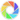• TA的每日心情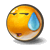擦汗
2018-4-10 15:18
• 签到天数: 447 天

[LV.9]以坛为家II

1742主题 13万积分

超级版主威望
562
贡献
29
金币
51888
钢镚
1422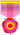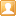发表于 2015-8-20 14:24:13 | 显示全部楼层 |阅读模式
 本帖最后由 破晓 于 2015-8-20 14:46 编辑 LMS  算法： LMS算法的更新迭代公式是：w(n+1) = w(n) + 2ηe * (n) x(n), 算法每次迭代计算仅需要 M + 1 次惩罚和M次加法计算 %%%%%%%%%%%%%%%%%%%%%%%%%%%%%%%%%%%% %LMS自适应滤波器性能分析 %%%%%%%%%%%%%%%%%%%%%%%%%%%%%%%%%%%%%% clear clc close all %%%%%%%%%%%%%%参数设置%%%%%%%%%%%%%%%%%%%%%%% w=3; % step_len=0.075;%步长 step_len=0.05;%步长 variance_v=0.01;%方差 repeat_times=20;%重复次数 iteration_times=500;%迭代次数 filt_len=2;%滤波器长度 delay=fix(filt_len/2)-1; delay1=1; %%%%%%%%%%%%%%%%%%%%变量及数组初始化%%%%%%%%%%%%%%%%%%%%%%%%%%%%%%%%%%%%%% Chan_factor=(1+cos(2*pi*([1:1:3]-2)/w))/2 Square_err=zeros(repeat_times,iteration_times); % Chan_factor1=[-0.195 0.95] Chan_factor1=[-0.195 0.95] X=zeros(filt_len,1); W1=[]; W2=[]; Wm1=[repeat_times,iteration_times]; Wm2=[repeat_times,iteration_times]; %%%%%%%%%%%%%外循环，重复做repeat_timws次实验，平均值%%%%%%%%%%%%%%%% for loop1=1:repeat_times%循环次数       %%%%%%%%%%%%%%内循环变量及数组初始化%%%%%%%%%%%%%%%%%%%%%%%% Source=zeros(iteration_times,1); W=zeros(filt_len,1); %%%%%%%%%%%%%内循环，做iteration_times次迭代%%%%%%%%%%%%%%%%%%%%%%%%%%%%    for i=1:iteration_times %%%%%%%%%%%%%%信源，产生等概率分布的正负1%%%%%%%%%%%%%%%%%%%%%%%%%%%%       if rand<0.5           Source(i)=-1;       else           Source(i)=1;       end    end %%%%%%%%%%%%%%信道，滤波和加噪声%%%%%%%%%%%%%%%%%%%%%%%%%%%%%%%%% Revdata=filter(Chan_factor1,1,Source)+randn(iteration_times,1)*sqrt(variance_v); %  Revdata=filter(Chan_factor,1,Source)+randn(iteration_times,1)*sqrt(variance_v); %    Revdata=filter(Chan_factor1,1,Source);     % RT=filter(Chan_factor1,1,Source); %%%%%%%%%%%%%%均衡器，基于LMS算法%%%%%%%%%%%%%%%%%%%%%%%%%%%%%%%%        for loop2=1:iteration_times-filt_len+1           %         U=Revdata(loop2:loop2+filt_len-1); %          y(n)=W'*U=W'*x(n),U=x(n)=输入 %          d为输出         U=Source(loop2:loop2+filt_len-1);         d=Revdata(loop2+1); %         d=Source(loop2+delay); %         X= x(3)=v(3)-a1*x(2)-a2*x(1);           %           d=RT(loop2+delay1); %         Y(loop2)=W'*U;         e=d-W'*U; %         e=d-W'*U;         W=W+step_len*U*e;                   Wm1(loop2,loop1)=W(1);         Wm2(loop2,loop1)=W(2); %         W1(loop2)=W(1); %         W2(loop2)=W(2);         Square_err(loop1,loop2)=e.^2;     end             end %%%%%%%%%%%%%%作图，显示结果%%%%%%%%%%%%%%%%%%%%%%%%%%%%%%%%%%%%% Square_err_mean=sum(Square_err(:,:))/repeat_times; % Wm2 W1=Wm1(:,repeat_times); W2=Wm2(:,repeat_times); meanW1=mean(Wm1'); meanW2=mean(Wm2'); Square_err; %semilogy(Square_err_mean); figure(1); plot(Square_err_mean); hold on; plot(Square_err(1,:),'r'); legend('20次重复试验平均误差','单次误差'); xlabel('迭代次数'); ylabel('均方误差'); title('步长为0.075，500次迭代均方误差变化曲线'); grid on; hold on; figure(2); plot(W1); hold on; plot(W2,'r'); hold on; plot(meanW1,'k'); hold on; plot(meanW2,'--k'); legend('W1','W2','20次重复试验W1','20次重复试验W2'); xlabel('迭代次数'); ylabel('抽头权值'); title('步长为0.075，500次迭代权值学习曲线'); grid on;复制代码 RLS 算法 %%%%%%%%%%%%%%%%%%%%%%%%%%%%%%%%%%%% %RLS自适应滤波器性能分析 %%%%%%%%%%%%%%%%%%%%%%%%%%%%%%%%%%%%%% clear all %clc %close all %%%%%%%%%%%%%%参数设置%%%%%%%%%%%%%%%%%%%%%%% w=2.9; % forget_factor=0.995; forget_factor=0.5; variance_v=0.001; repeat_times=20; iteration_times=500; filt_len=2; delay=fix(filt_len/2)-1; %%%%%%%%%%%%%%%%%%%%变量及数组初始化%%%%%%%%%%%%%%%%%%%%%%%%%%%%%%%%%%%%%% Chan_factor=(1+cos(2*pi*([1:1:3]-2)/w))/2; Chan_factor1=[-0.195,0.95] Square_err=zeros(repeat_times,iteration_times); W1=[]; W2=[]; Wm1=[repeat_times,iteration_times]; Wm2=[repeat_times,iteration_times]; %%%%%%%%%%%%%外循环，重复做repeat_times次实验，平均值%%%%%%%%%%%%%%%% for loop1=1:repeat_times             %%%%%%%%%%%%%%内循环变量及数组初始化%%%%%%%%%%%%%%%%%%%%%%%% Source=zeros(iteration_times,1); W=zeros(filt_len,1); P=eye(filt_len); Pi=zeros(filt_len,1); K=zeros(filt_len,1); %%%%%%%%%%%%%内循环，做iteration_times次迭代%%%%%%%%%%%%%%%%%%%%%%%%%%%%    for i=1:iteration_times %%%%%%%%%%%%%%信源，产生等概率分布的正负1%%%%%%%%%%%%%%%%%%%%%%%%%%%%       if rand<0.5           Source(i)=-1;       else           Source(i)=1;       end    end %%%%%%%%%%%%%%信道，滤波和加噪声%%%%%%%%%%%%%%%%%%%%%%%%%%%%%%%%%    Revdata=filter(Chan_factor1,1,Source)+randn(iteration_times,1)*sqrt(variance_v); %%%%%%%%%%%%%%均衡器，基于RLS算法%%%%%%%%%%%%%%%%%%%%%%%%%%%%%%%%        for loop2=1:iteration_times-filt_len+1 %         U=Revdata(loop2:loop2+filt_len-1); %         d=Source(loop2+delay);         U=Source(loop2:loop2+filt_len-1);         d=Revdata(loop2+1);         Pi=P*U;         K=Pi/(forget_factor+U'*Pi);         e=d-W'*U;         W=W+K*e;         P=P/forget_factor-K*U'*P/forget_factor;                   Wm1(loop2,loop1)=W(1);         Wm2(loop2,loop1)=W(2);         Square_err(loop1,loop2)=e.^2;     end end %%%%%%%%%%%%%%作图，显示结果%%%%%%%%%%%%%%%%%%%%%%%%%%%%%%%%%%%%% Square_err_mean=sum(Square_err(:,:))/repeat_times; W1=Wm1(:,repeat_times) W2=Wm2(:,repeat_times) meanW1=mean(Wm1'); meanW2=mean(Wm2'); %semilogy(Square_err_mean); figure(1); plot(Square_err_mean); hold on; plot(Square_err(1,:),'r'); legend('20次重复试验平均误差','单次误差'); xlabel('迭代次数'); ylabel('均方误差'); title('遗忘因子为0.8，500次迭代均方误差变化曲线'); grid on; hold on; plot(Square_err_mean); hold off; figure(2); plot(W1); hold on; plot(W2,'r'); hold on; plot(meanW1,'k'); hold on; plot(meanW2,'--k'); legend('W1','W2','20次重复试验W1','20次重复试验W2'); xlabel('迭代次数'); ylabel('抽头权值'); title('遗忘因子为0.8，500次迭代权值学习曲线'); grid on;复制代码
守望者AIR技术交流社区（www.airmyth.com）
回复
• TA的每日心情擦汗
2018-4-10 15:18
• 签到天数: 447 天

[LV.9]以坛为家II

1742主题 13万积分

超级版主威望
562
贡献
29
金币
51888
钢镚
1422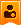楼主| 发表于 2015-8-20 18:08:30 | 显示全部楼层
 #include "math.h"    #include "stdio.h"   int     N=5;                           /* FIR滤波器阶数N */   float   u=0.001;                       /* 自适应步长u */   float   pi=3.1415926;   int     i,j;   float   x;                       float   y;   float   d;   float   e;   float   X;   float   W;   void main()   {          for (i=0;i<504;i++)                /* 输入信号为正弦 */       {            x[i]=sin((9*i-36)*pi/180);         }                                                      for (i=0;i<500;i++)                /* 期望信号等于输入信号 */         {            d[i]=x[i+N-1];         }              for (i=0;i
守望者AIR技术交流社区（www.airmyth.com）
回复 支持 反对
 本版积分规则 回帖后跳转到最后一页
﻿
关闭

## 站长推荐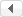/4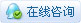|手机版|Archiver|网站地图|小黑屋|守望者 ( 京ICP备14061876号GMT+8, 2021-4-23 03:34 , Processed in 0.041108 second(s), 30 queries .守望者AIR技术交流社区 本站成立于 2014年12月31日